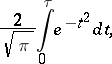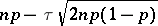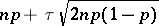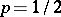# Gauss-Laplace distribution

(diff) ← Older revision | Latest revision (diff) | Newer revision → (diff)

One of the names given to the normal distribution which, together with other names such as Gauss law, Gaussian distribution, second law of Laplace, Laplace–Gauss distribution, etc., connects the discovery and the first applications of this distribution to various problems in probability theory with the names of C.F. Gauss and P. Laplace. Gauss (1809) and Laplace (1812) introduced the normal distribution in connection with their studies on the theory of errors and method of least squares (cf. Errors, theory of; Least squares, method of). Thus, in the theory of (observational) errors, developed by Gauss for problems in astronomy and theoretical geodesy, the probability density of random errors is given by the expression(cf. Gauss law). In addition, Laplace obtained the integral (the Laplace function)which gives the approximate value (for large) of the probability that the number of successful outcomes inBernoulli trials with a probability of successlies in betweenand(the so-called limit formula of Laplace). At an earlier date (1733), the relation in which the normal distribution appears as the limit form of the binomial distribution (with) had been discovered by A. de Moivre.

How to Cite This Entry:
Gauss-Laplace distribution. Encyclopedia of Mathematics. URL: http://encyclopediaofmath.org/index.php?title=Gauss-Laplace_distribution&oldid=13322
This article was adapted from an original article by A.V. Prokhorov (originator), which appeared in Encyclopedia of Mathematics - ISBN 1402006098. See original article